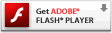# Interactive Generative Art Series – 07 – multiple linesTo make it more interesting and give more depth and texture to the display, I wanted to have more lines than one, so I’m now going with two for starters. Eventually I’d like to get up to a dozen or maybe even have them randomly self populate and die over time. Adding one more isn’t a whole lot of code, but it did require a bit of rearranging. I created a sprite container for each line, and then basically doubled any var used by one line for the other. When calculating the position for the second line I added a bit of randomness, so it wouldn’t be drawn in the exact same position of the first line. Plus, when drawing the second line I adjust the alpha/transparency a bit and the line width. A big issues that I notice right away is that the memory noticeably begins to suffer after a bit of drawing. After some investigation I determined it is (at least in part) the fact that the lines now have to blend with the lines below them with transparency. If I set the alpha properties in the lineGradientStyle to 1 and 1 the performance is much better. Any ideas of how to fix this? I’m guessing I should start investigating bitmap data.

### 07 multiline, play here

Please visit the blog article to view this interactive flash content. Flash plug-in required:### actionscript source code

[cc lang=”actionscript”]
var ball:Sprite = new Sprite();
ball.graphics.beginFill(0x000000, 1);
ball.graphics.drawCircle(0, 0, 10);
ball.graphics.endFill();
var ball2:Sprite = new Sprite();
ball2.graphics.beginFill(0x000000, 1);
ball2.graphics.drawCircle(0, 0, 5);
ball2.graphics.endFill();

var balllines:Sprite = new Sprite();
var ball2lines:Sprite = new Sprite();

var anchor:Sprite = new Sprite();

anchor.graphics.beginFill(0x333333, .6);
anchor.graphics.drawCircle(0, 0, 12);
anchor.graphics.endFill();

var div:Number = .1;
var line_max_width:Number = 64;
var line_min_width:Number = 1;
var line_width:Number = randomRange(line_min_width, line_max_width);
var line_width_velocity:Number = 0;
var dampen:Number = 0.95;

var ballax:Number = 0;
var ballay:Number = 0;
var oldx:Number = ball.x;
var oldy:Number = ball.y;
var ball2ax:Number = 0;
var ball2ay:Number = 0;
var old2x:Number = ball2.x;
var old2y:Number = ball2.y;

var anchorvx:Number = 0;
var anchorvy:Number = 0;

anchor.x = stage.stageWidth/2;
anchor.y = stage.stageHeight/2;
ball2.x = randomRangeAxis(10);

var colors:Object = new Object();
colors.r = 255;
colors.g = 255;
colors.b = 255;
colors.rv = 0;
colors.gv = 0;
colors.bv = 0;
colors.rmin = 150; //0
colors.rmax = 250; //100
colors.gmin = 0; //100
colors.gmax = 150; //200
colors.bmin = 0; //150
colors.bmax = 100; //250
colors.rate_of_change = 12;
var color_first:Number = 0xFFFFFF;
var color_second:Number = rgb2hex(colors.r, colors.g, colors.b);

function loop () {

oldx = ball.x;
oldy = ball.y;
old2x = ball2.x;
old2y = ball2.y;

anchorvx += randomRangeAxis(10);
anchorvy += randomRangeAxis(10);

anchor.x += anchorvx;
anchor.y += anchorvy;

anchorvx *= dampen;
anchorvy *= dampen;

if(anchor.x > stage.stageWidth) {
anchor.x = 0 – anchor.width;
}
else if(anchor.x < 0 - anchor.width) { anchor.x = stage.stageWidth; } if(anchor.y > stage.stageHeight) {
anchor.y = 0 – anchor.height;
}
else if(anchor.y < 0 - anchor.height) { anchor.y = stage.stageHeight; } ball.x -= ballax = (ballax + (ball.x - anchor.x) * div) * .9; ball.y -= ballay = (ballay + (ball.y - anchor.y) * div) * .9; ball2.x -= ball2ax = (ball2ax + (ball2.x - (anchor.x + randomRangeAxis(line_width * 2))) * div) * .9; ball2.y -= ball2ay = (ball2ay + (ball2.y - (anchor.y + randomRangeAxis(line_width * 2))) * div) * .9; line_width_velocity += randomRangeAxis(1); line_width += line_width_velocity; line_width_velocity *= dampen; if(line_width > line_max_width) {
line_width = line_max_width;
line_width_velocity = 0;
}
else if (line_width < line_min_width) { line_width = line_min_width; line_width_velocity = 0; } color_step(); color_first = color_second; color_second = rgb2hex(colors.r, colors.g, colors.b); var dx:Number = ball.x - oldx; var dy:Number = ball.y - oldy; balllines.graphics.lineStyle(line_width, color_first, (line_width+100-line_max_width)/100, true, LineScaleMode.NONE, CapsStyle.NONE); gradientBoxMatrix.createGradientBox(Math.abs(dx), Math.abs(dy), Math.atan2(dy,dx), Math.min(oldx, ball.x), Math.min(oldy, ball.y)); balllines.graphics.lineGradientStyle(GradientType.LINEAR, [color_first, color_second], [(line_width+100-line_max_width)/100,(line_width+100-line_max_width)/100], [0, 255], gradientBoxMatrix); balllines.graphics.lineTo(ball.x, ball.y); dx = ball2.x - old2x; dy = ball2.y - old2y; ball2lines.graphics.lineStyle(line_width/2, color_first, (line_width+100-line_max_width)/100, true, LineScaleMode.NONE, CapsStyle.NONE); gradientBoxMatrix.createGradientBox(Math.abs(dx), Math.abs(dy), Math.atan2(dy,dx), Math.min(old2x, ball2.x), Math.min(old2y, ball2.y)); ball2lines.graphics.lineGradientStyle(GradientType.LINEAR, [color_first + 3072, color_second + 3072], [((line_width/2)+100-line_max_width)/100,((line_width/2)+100-line_max_width)/100], [0, 255], gradientBoxMatrix); ball2lines.graphics.lineTo(ball2.x, ball2.y); } setInterval(loop, 1000/30); function rgb2hex(r:Number, g:Number, b:Number):Number { return(r<<16 | g<<8 | b); } function color_step(){ colors.rv += randomRangeAxis(colors.rate_of_change); colors.r += colors.rv; colors.rv *= dampen; if (colors.r > colors.rmax) {
colors.r = colors.rmax;
} else if (colors.r < colors.rmin){ colors.r = colors.rmin; } colors.gv += randomRangeAxis(colors.rate_of_change); colors.g += colors.gv; colors.gv *= dampen; if (colors.g > colors.gmax) {
colors.g = colors.gmax;
} else if (colors.g < colors.gmin){ colors.g = colors.gmin; } colors.bv += randomRangeAxis(colors.rate_of_change); colors.b += colors.bv; colors.bv *= dampen; if (colors.b > colors.bmax) {
colors.b = colors.bmax;
} else if (colors.b < colors.bmin){ colors.b = colors.bmin; } } //random number between min and max function randomRange(max:Number, min:Number = 0):Number { return Math.random() * (max - min) + min; } //random number range centered at 0 with the specified max, randomRange(-max, max) function randomRangeAxis(max:Number, axis:Number = 0):Number { return Math.random() * (max * 2) - max + axis; } [/cc]

1.Nico says: